# Initialize Clustering Model (K-Means) VI

LabVIEW 2018 Analytics and Machine Learning Toolkit Help

Edition Date: July 2018

Part Number: 377059B-01

»View Product InfoDownload Help (Windows Only)

Owning Palette: Clustering VIs

Requires: Analytics and Machine Learning Toolkit

Initializes the hyperparameters of the K-Means algorithm. You can either directly set the hyperparameters or specify multiple values for each hyperparameter. If you specify multiple values for each hyperparameter, the Train Clustering Model VI uses grid search to find the optimal set of hyperparameters.

Examples

Use the pull-down menu to select an instance of this VI.

 Select an instance Set ParametersSearch Parameters

## Set Parameters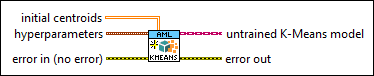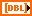initial centroids specifies the initial centroids. This input is valid only if initial method is Custom.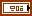hyperparameters specifies the hyperparameters for the K-Means model.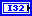number of clusters specifies the number of clusters in the K-Means model. The default is 3.tolerance specifies the tolerance for the stopping criteria. The default is 0.00001.max iteration specifies the maximum number of optimization iterations for the stopping criteria. The default is 50. The model fitting stops if the number of optimization iterations reaches max iteration.initial method specifies the method to select initial centroids.

 0 K-Means++ (default)—Selects initial centroids in a way that can always speed up convergence. 1 Random—Randomly selects a specific number of observations from the data and uses them as the initial centroids. This method is also called the Forgy method. 2 Custom—Directly specifies the initial centroids using the initial centroids input.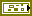error in describes error conditions that occur before this node runs. This input provides standard error in functionality.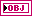untrained K-Means model returns the initialized K-Means model for training.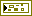error out contains error information. This output provides standard error out functionality.

## Search Parameters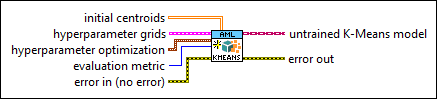initial centroids specifies the initial centroids. This input is valid only if initial method is Custom.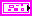hyperparameter grids specifies multiple values for each hyperparameter.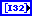number of clusters specifies the number of clusters in the K-Means model.attempts specifies the number of times that the Train Clustering Model VI trains the model with different hyperparameter combinations.tolerance specifies the tolerance for the stopping criteria.max iteration specifies the maximum number of optimization iterations for the stopping criteria. The model fitting stops if the number of optimization iterations reaches max iteration.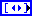initial method specifies the initial method.

 0 K-Means++ (default)—Selects initial centroids in a way that can always speed up convergence. 1 Random—Randomly selects a specific number of observations from the data and uses them as the initial centroids. This method is also called the Forgy method. 2 Custom—Directly specifies the initial centroids using the initial centroids input.hyperparameter optimization specifies the method of optimization to determine the optimal hyperparameter settings.hyperparameter search method specifies the method to search for the optimal set of hyperparameters.

 0 Exhaustive search (default)—Tests all possible hyperparameter combinations in the training process. This method is reliable but time-consuming. 1 Random search—Tests a random subset of the hyperparameter combinations in the training process. This method is faster.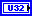number of searchings specifies the number of hyperparameter combinations that this VI selects to train the model. The default is 1. This input is valid only if hyperparameter search method is Random search.evaluation metric specifies the criterion to evaluate the trained clustering model.

 0 Davies Bouldin Index (default)—Evaluates the clustering model using the Davies Bouldin Index metric. The lower the value of metric, the better the compactness and separation of the clustering model. 1 Dunn Index—Evaluates the clustering model using the Dunn Index metric. The higher the value of metric, the better the compactness and separation of the clustering model. 2 Jaccard Index—Evaluates the clustering model using the Jaccard Index metric. The lower the value of metric, the better the separation of the clustering model. 3 Rand Index—Evaluates the clustering model using the Rand Index metric. The lower the value of metric, the better the separation of the clustering model.error in describes error conditions that occur before this node runs. This input provides standard error in functionality.untrained K-Means model returns the initialized K-Means model for training.error out contains error information. This output provides standard error out functionality.

## Examples

Refer to the following VIs for examples of using the Initialize Clustering Model (K-Means) VI:

• Clustering (Set Parameters, Training) VI: labview\examples\AML\Clustering
• Clustering (Search Parameters, Training) VI: labview\examples\AML\Clustering